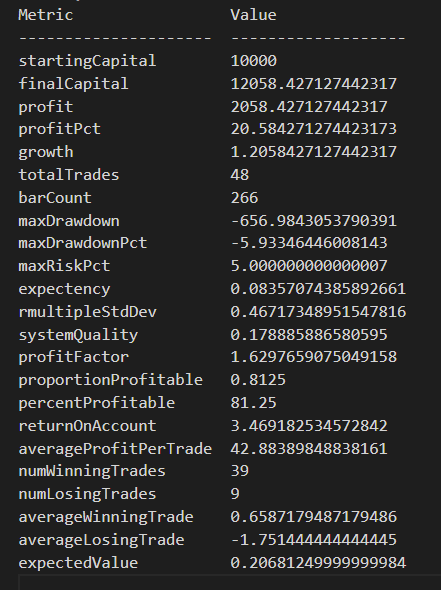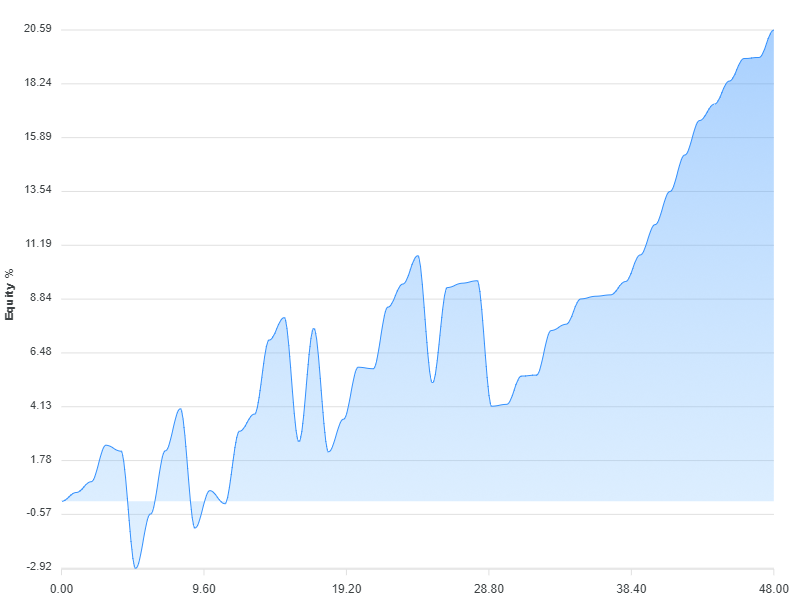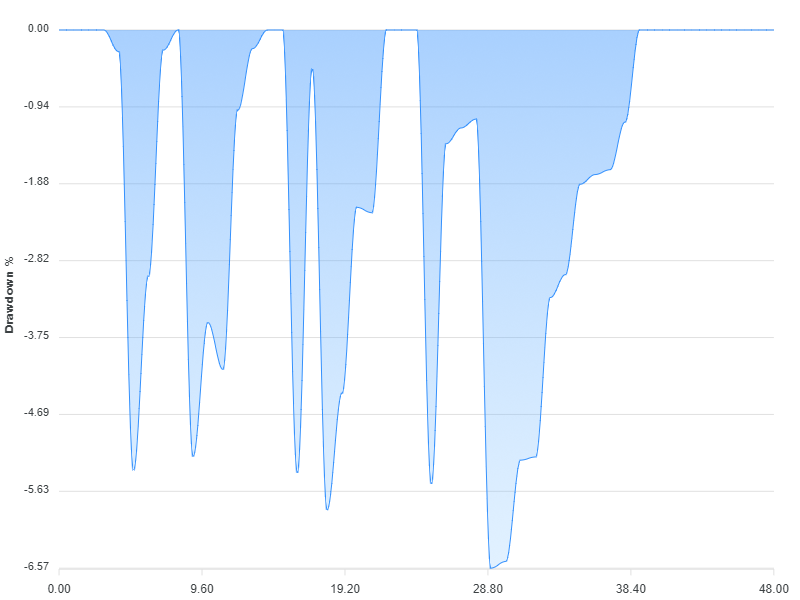# An API for backtesting trading strategies in JavaScript and TypeScript.Grademark Last update: Mar 18, 2023

Toolkit for algo trading and backtesting in JavaScript and TypeScript.

This API builds on Data-Forge and is best used from Data-Forge Notebook (making it easy to plot charts and visualize).

Check out the release notes to see updates and breaking changes.

Please see what this looks like in the Grademark first example and the unit tests in this repo.

Love this? Please star this repo!

## First example

From the Grademark first example here's some example output. Click to see the first example as a notebook.

Analysis of a sequence of trades looks like this:Here's a chart that visualizes the equity curve for the example strategy:Here's another chart, this one is a visualization of the drawdown for the example strategy:## Pre-requisites

• Make sure your data is sorted in forward chronological order.

## Data format

Your data needs to be loaded into memory in the following format:

```interface IBar {
time: Date;
open: number;
high: number;
low: number;
close: number;
}

## Features

• Define a trading strategy with entry and exit rules.
• Backtest a trading strategy on a single financial instrument.
• Apply custom indicators to your input data series.
• Specify lookback period.
• Built-in intrabar stop loss.
• Compute and plot equity curve and drawdown charts.
• Throughly covered by automated tests.
• Calculation of risk and rmultiples.
• Intrabar profit target.
• Intrabar trailing stop loss.
• Conditional buy on price level (intrabar).
• Monte carlo simulation.
• Multiple parameter optimization based on permutations of parameters (using grid search and hill-climb algorithms).
• Walk forward optimization and backtesting.
• Plot a chart of trailing stop loss.
• Short selling.

Data-Forge Notebook comes with example JavaScript notebooks that demonstrate many of these features.

If you need help with new features please reach out!

## Maybe coming later

• Support for precise decimal numbers.
• Fees.
• Slippage.
• Position sizing.
• Testing multiple instruments / portfolio simulation / ranking instruments.
• Market filters.

## Usage

Instructions here are for JavaScript, but this library is written in TypeScript and so it can also be used from TypeScript.

### Installation

``````npm install --save grademark
``````

### Import modules

```const dataForge = require('data-forge');
require('data-forge-indicators'); // For the moving average indicator.
require('data-forge-plot'); // For rendering charts.
const { backtest, analyze, computeEquityCurve, computeDrawdown } = require('grademark');```

Use Data-Forge to load and prep your data, make sure your data is sorted in forward chronological order.

This example loads a CSV file, but feel free to load your data from REST API, database or wherever you want!

```let inputSeries = dataForge.readFileSync("STW.csv")
.parseCSV()
.parseDates("date", "D/MM/YYYY")
.parseFloats(["open", "high", "low", "close", "volume"])
.setIndex("date") // Index so we can later merge on date.
.renameSeries({ date: "time" });```

The example data file is available in the example repo.

```const movingAverage = inputSeries
.deflate(bar => bar.close)          // Extract closing price series.
.sma(30);                           // 30 day moving average.

inputSeries = inputSeries
.withSeries("sma", movingAverage)   // Integrate moving average into data, indexed on date.
.skip(30)                           // Skip blank sma entries.```

### Create a strategy

This is a very simple and very naive mean reversion strategy:

```const strategy = {
entryRule: (enterPosition, args) => {
if (args.bar.close < args.bar.sma) { // Buy when price is below average.
enterPosition({ direction: "long" }); // Long is default, pass in "short" to short sell.
}
},

exitRule: (exitPosition, args) => {
if (args.bar.close > args.bar.sma) {
exitPosition(); // Sell when price is above average.
}
},

stopLoss: args => { // Optional intrabar stop loss.
return args.entryPrice * (5/100); // Stop out on 5% loss from entry price.
},
};```

### Running a backtest

Backtest your strategy, then compute and print metrics:

```const trades = backtest(strategy, inputSeries)

const startingCapital = 10000;
console.log(analysis);```

### Visualizing the results

Use Data-Forge Plot to visualize the equity curve and drawdown chart from your trading strategy:

```computeEquityCurve(trades)
.plot()
.renderImage("output/my-equity-curve.png");

.plot()
.renderImage("output/my-drawdown.png");```